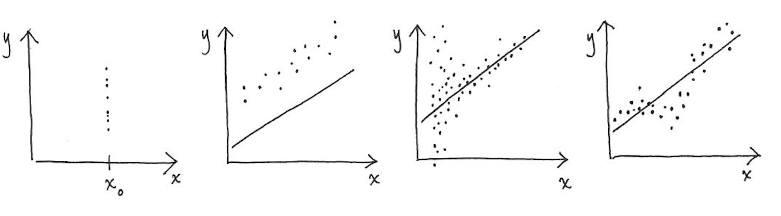top of page
All Posts: Blog2

# [Math Evenings] Bivariate Linear Models: Basics & Specifics

Updated: Apr 3, 2021

Mathematics often grants us the power to predict the future, and modelling is perhaps the epitome of such a characteristic. Within mathematical models, linear models are one of the most widely used models.Examples of when 2nd, 3rd, 4th, 5th Conditions are violated, from left to right

Note: The 5th diagram is an example of serial correlation. There is an area where most data is above the line, and there is also an area where most is below the line. This is an example of serial correlation.

I put in this diagram, because they nicely illustrate why the conditions are necessary without putting in much words. It was not because I was too lazy to write the reasons. Definitly not.

10 views

See All
bottom of page